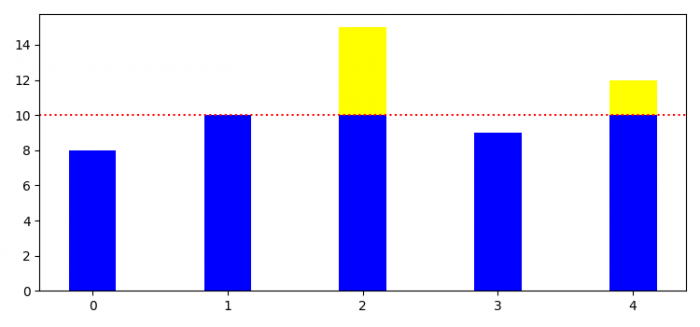# How to create a Matplotlib bar chart with a threshold line?

To create a Matplotlib bar chart with a threshold line, we have to use axhline() method.

## Steps

• Set the figure size and adjust the padding between and around the subplots.
• Initialize a variable, threshold.
• Make lists for bars values.
• Get the below and above bar values based on the threshold value.
• Create a figure and a set of subplots using subplots() method.
• Plot bars with x, a_threshold and b_threshold values.
• Add a horizontal line across the axis using axhline() method.
• To display the figure, use show() method.

## Example

import numpy as np
import matplotlib.pyplot as plt

plt.rcParams["figure.figsize"] = [7.50, 3.50]
plt.rcParams["figure.autolayout"] = True
threshold = 10
values = np.array([8.0, 10.0, 15.0, 9.0, 12.0])
x = range(len(values))

a_threshold = np.maximum(values - threshold, 0)
b_threshold = np.minimum(values, threshold)

fig, ax = plt.subplots()
ax.bar(x, b_threshold, 0.35, color="blue")
ax.bar(x, a_threshold, 0.35, color="yellow", bottom=b_threshold)

plt.axhline(threshold, color='red', ls='dotted')

plt.show()

## Output